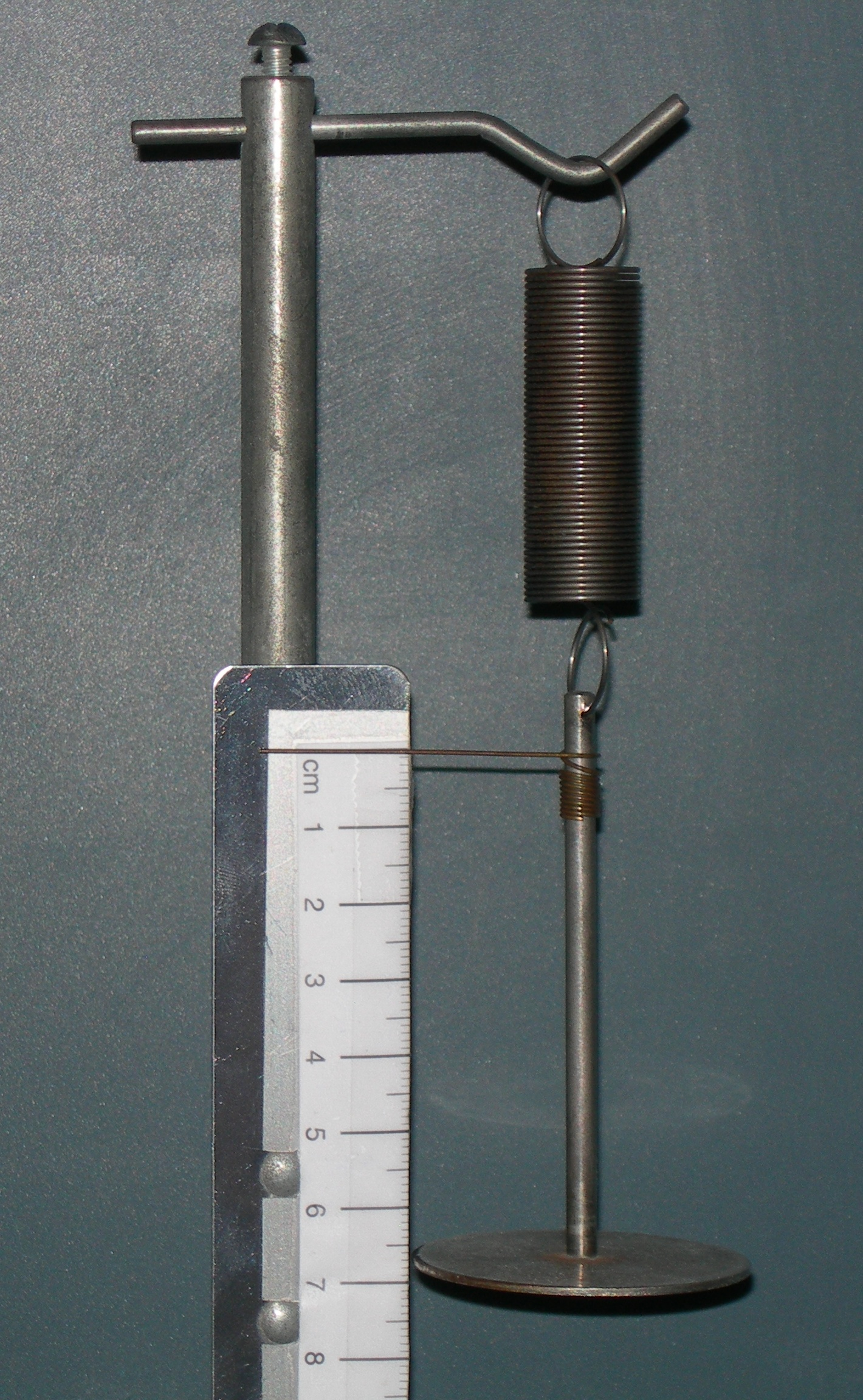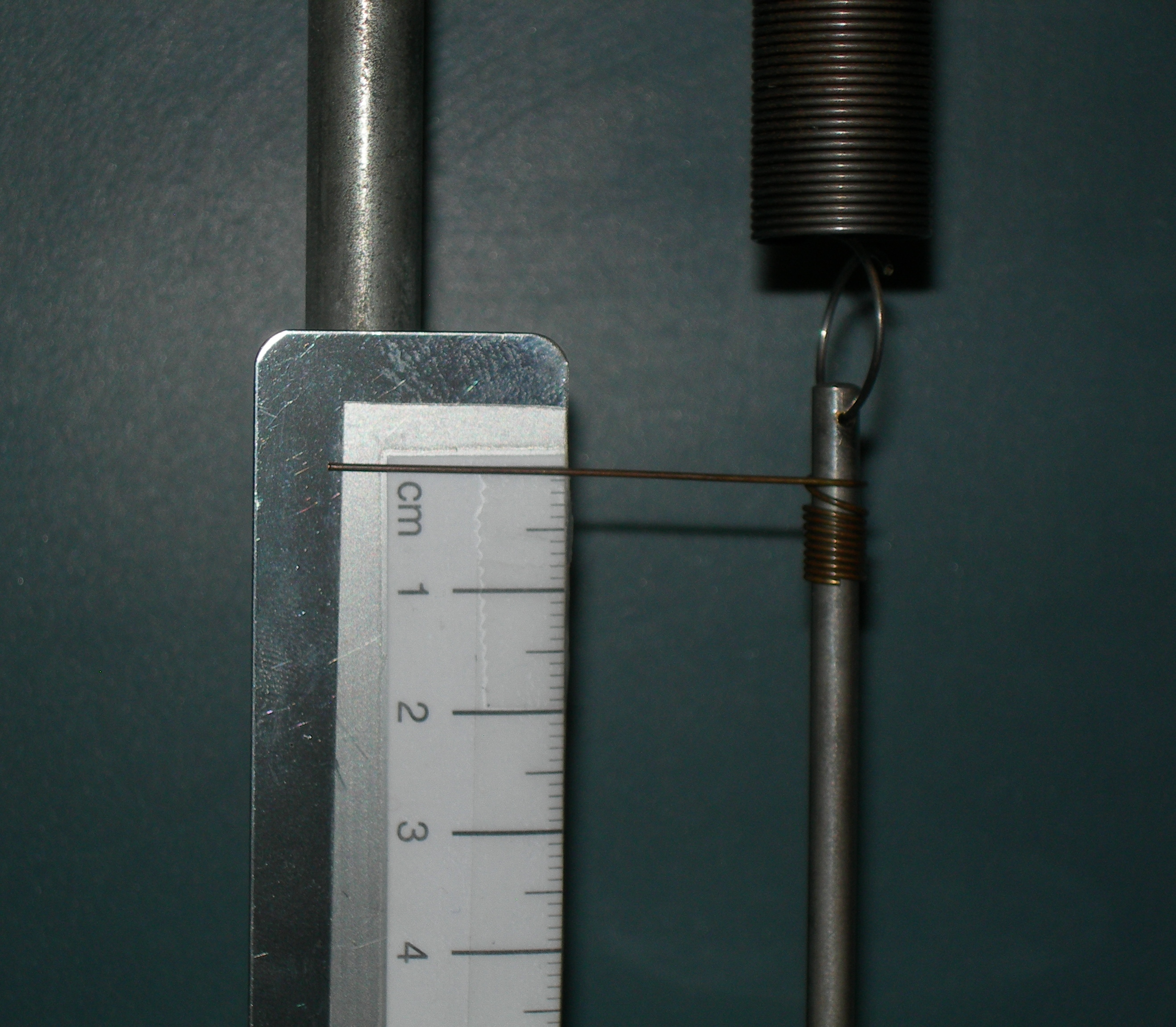Cycle I Physics
John Dewey High School
Mr. Klimetz
Hooke's Law and Its Application to Springs
Laboratory No. 4
Introduction. Largely as a consequence of the efforts of English physicist Robert Hooke, it is well-established that the extension produced in an elastic body experiencing tension is directly proportional to the magnitude of the applied force. This statement is, in fact, the definition of elastic behavior and is the criterion which must be met for an object to be considered as purely elastic. This relationship however, is valid only for objects which have not been subjected to forces at which permanent deformation results, namely, the elastic limit. Simply stated, all objects exhibit elastic behavior but only to a certain extent. Every object will ultimately experience permanent deformation (permanent strain) once the applied forces become so great as to fundamentally and irrevocably change the internal architecture of the object by overcoming attractive interatomic and intermolecular forces. At force levels in excess of the elastic limit, objects will most likely experience failure, the physical separation (or breakdown) of the object into two (or more) individual objects.Hooke's Law Apparatus2 helical springs1-10 gram slotted mass2-20 gram slotted masses4-50 gram slotted masses1-100 gram slotted masspencils with eraserscalculatorgraph paper
Methods Employed in the Determination of the Elastic Constant of a Helical Spring
Mathematical Expression of Hooke's Law.  The relationship between an applied tensile force to an object and its observed elongation can be simply stated as
Force = Elasticity Constant x Elongation
where force is expressed in Newtons, elongation is expressed in meters, and the elasticity constant is therefore expressed in Newtons/meter. The elasticity constant, which is a constant of proportionality relating the amount of applied force required to produce a specific elongation, is specific to a particular material or object. Weak (or easily stretchable) bodies possess a low elasticity constant whereas strong (or resistant) bodies possess a high elasticity constant.
Objectives. The purpose of this laboratory activity is to reaffirm Hooke's Law as it applies to the selective force loading of two helical (coiled) springs of differing elasticity. The force loading will be accomplished through the established incremental addition of mass to each spring which is vertically suspended from a fixed position. Since mass also possesses weight (a force), the addition of greater amounts of mass hence translates into greater amounts of force. As more weight is applied to a spring, the elongation of the spring will increase. Provided that a precise measurement of incremental elongation is made with each successive loading and as long as the elastic limit of the spring is not reached, the applied force should be directly proportional to the elongation. This relationship is to be tested both mathematically as well as graphically.
Materials.
Procedure.1.  Assemble the Hooke's Law Apparatus as instructed (see picture at right). Make sure that the wire pointer attached to the mass caddy is horizontal and set at the zero centimeter mark on the scale attached to the upright of the apparatus. (The scale is adjusted merely by sliding it either up or down the upright.) Also make sure that the caddy is freely suspended, and does not contact the apparatus in any way.2.  Add masses incrementally to the spring caddy such that the total amount of mass suspended is20g, 30g, 40g, 50g, 70g, 90g, 100g, 130g, 160g, 190g, 220g, 250g3. Observe each elongation produced by each mass increment by noting the position of the wire pointer with respect to the vertical metric scale. Record your elongation values to the level of precision permitted by the scale (0.1 cm). Enter all observations in the data table provided.4.  Convert all masses to kilograms, calculate their respective weights, and record.5.  Convert all elongations to meters and record.6.  Divide weight by elongation (in meters) and record. This is your elastic constant.
Data Analysis.Create a Force versus Elongation graph of your measurements in which force values are placed along the ordinate (y-axis) and elongation values are placed along the abscissa (x-axis). After all twelve data points for each spring have been plotted, sketch-in a "best-fit" line which averages (or normalizes) the points to a single linear function. Then determine the slope of your best fit lines. The slope of each line is equal to the average elasticity constant for each respective spring. Check this value by calculating the average of the elasticity constants determined numerically and listed in the data table.
Questions.1.  How do the spring constants you have determined relate to the behavior of the springs? Does the physical "feel" of the springs when stretched between your fingers support your determinations? How do the spring constants determined mathematically compare to those derived by graphical means?2.  Drawing best-fit lines such as the ones we have just produced is a form of data interpolation (or estimating). Write an equation that expresses the relationship between applied force and elongation for each of your best-fit lines.3.  Extrapolation is the process whereby we extend an established numerical relationship between two variables beyond the limits of our observed and recorded data. Explain why extrapolation would not be useful (and wise) in this particular situation.5.  The cables of a suspension bridge as well as the elements of many man-made structures are designed to behave elastically. Briefly explain why it is absolutely necessary that such elements function elastically.4.  Briefly explain the meaning and significance of the elastic limit and why it should be avoided.6.  Hooke's Law is a direct relationship. Briefly explain what this means and how your data illustrate this.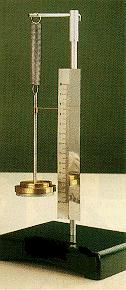Hooke's
Law
Apparatus
Wire Pointer
Spring
Masses
Scale
Stand
Clamp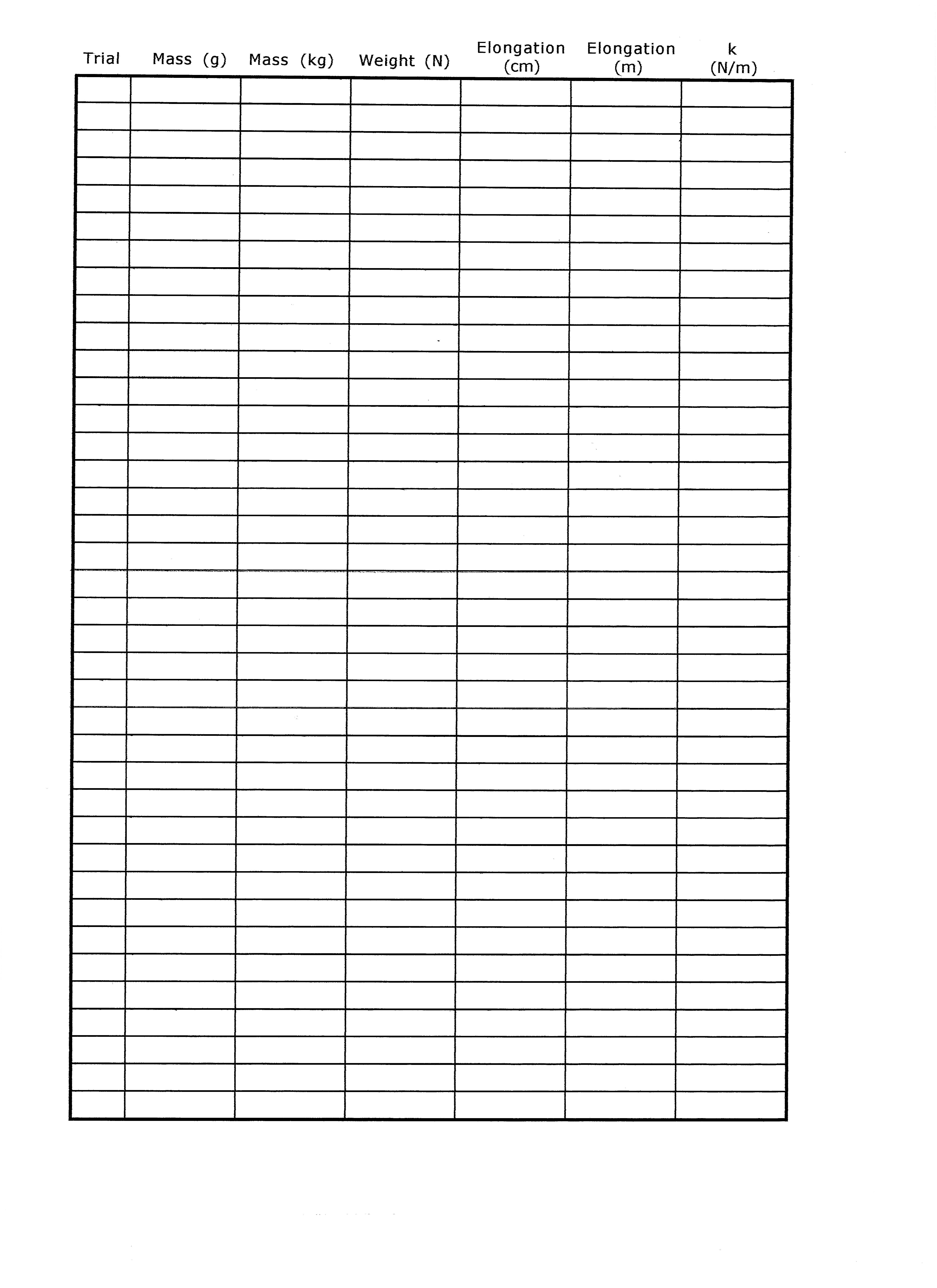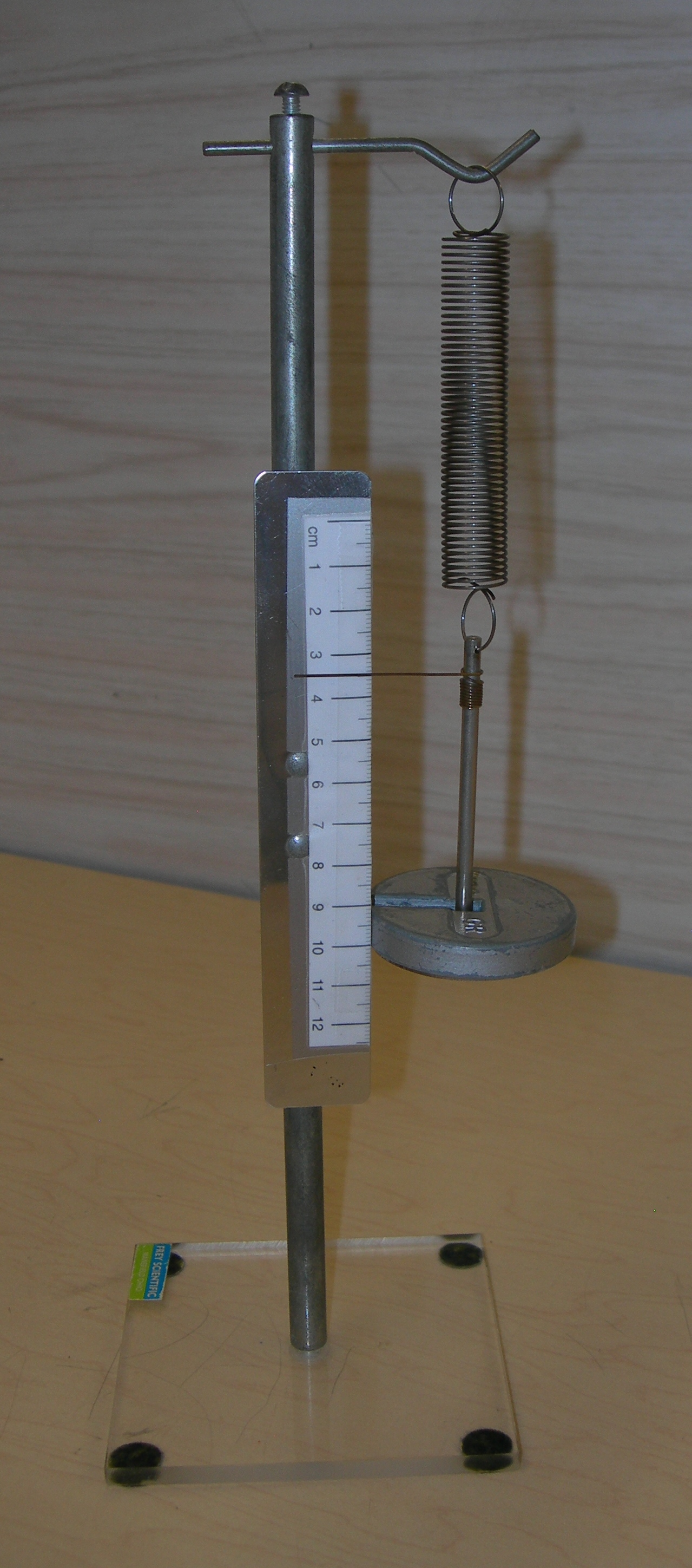JDHS Hooke's Law Apparatus
SpringStand
Scale
Wire Pointer
Mass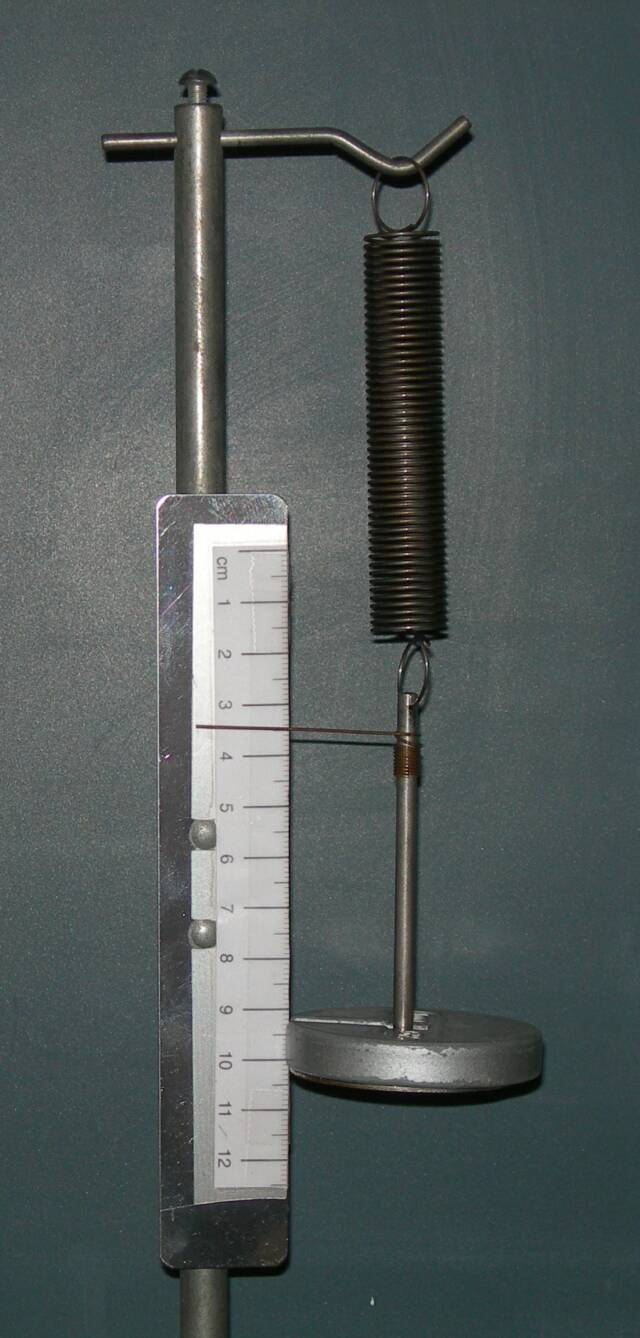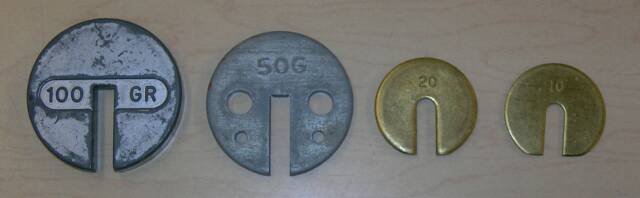Slotted Mass Coins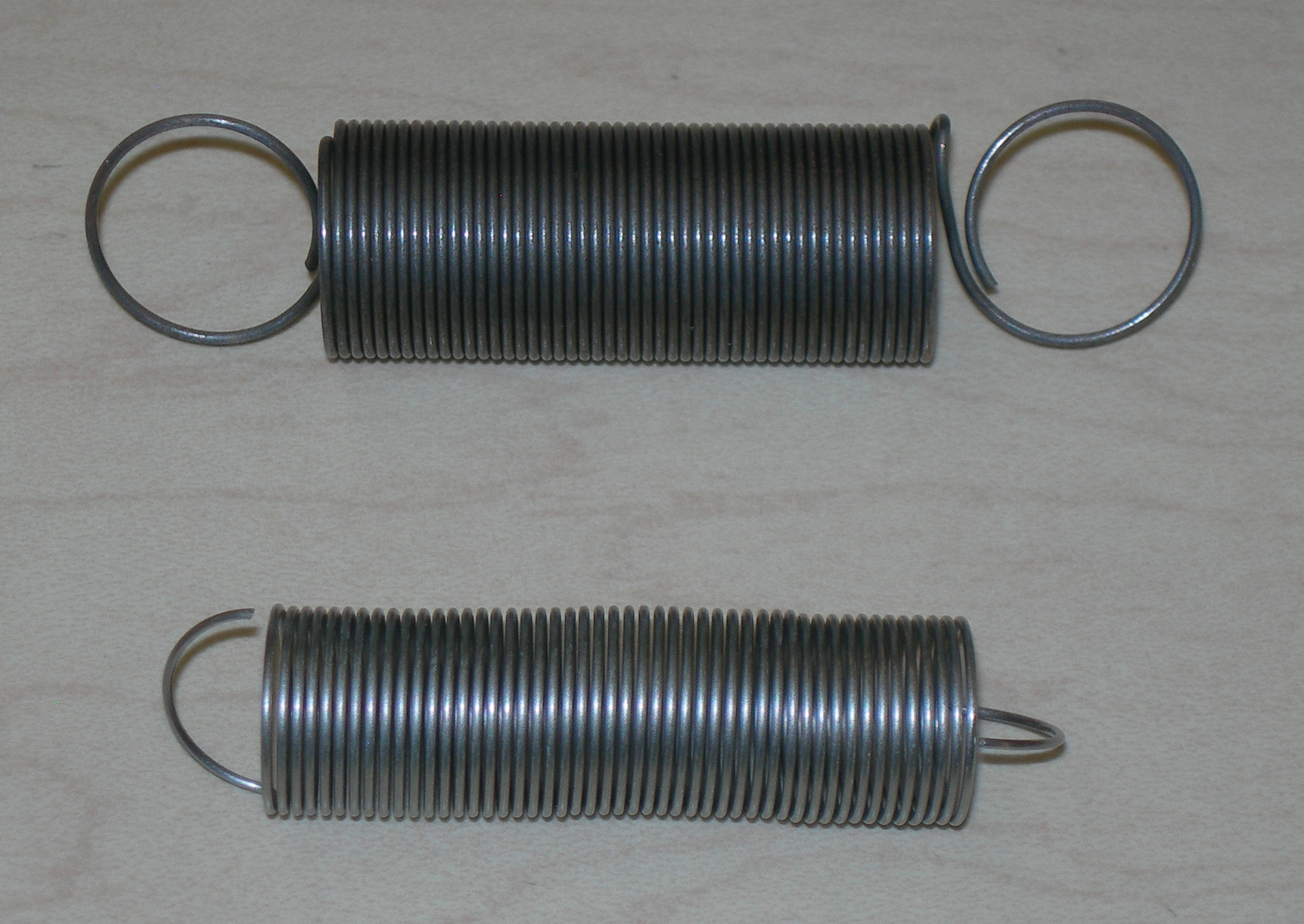Helical Springs
Dark Spring
Silver Spring